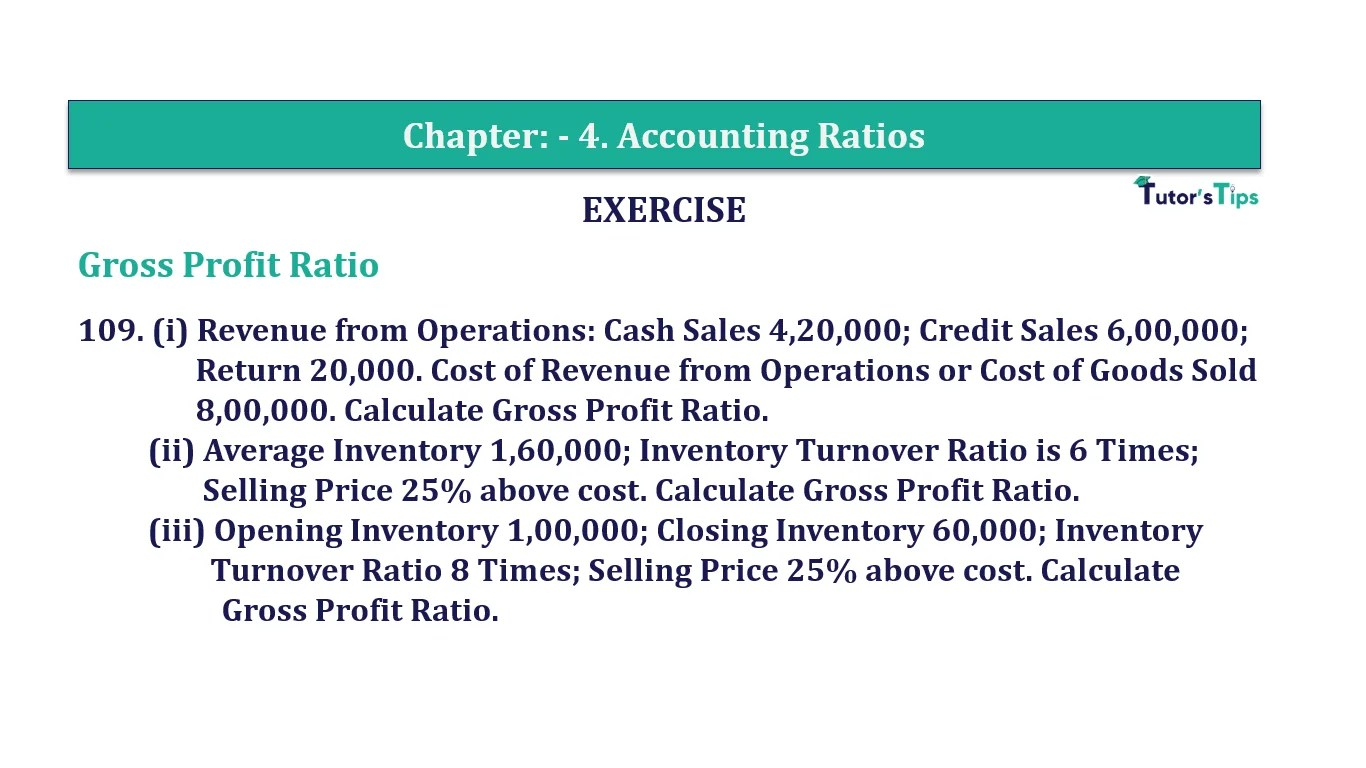# Question 109 Chapter 4 of +2-B – T.S. Grewal 12 ClassQuestion No. 109- Chapter No.4 - T.S. Grewal +2 Book Part B

Question 109 Chapter 4 of +2-B

Gross Profit Ratio

109. (i) Revenue from Operations: Cash Sales 4,20,000; Credit Sales 6,00,000;
Return 20,000. Cost of Revenue from Operations or Cost of Goods Sold
8,00,000. Calculate Gross Profit Ratio.
(ii) Average Inventory 1,60,000; Inventory Turnover Ratio is 6 Times;
Selling Price 25% above cost. Calculate Gross Profit Ratio.
(iii) Opening Inventory 1,00,000; Closing Inventory 60,000; Inventory
Turnover Ratio 8 Times; Selling Price 25% above cost. Calculate
Gross Profit Ratio.

### The solution of Question 109 Chapter 4 of +2-B: –

Case I :

 Net Sales = Cash Sales + Credit Sales – Sales Return = Rs. 4,20,000 + Rs. 6,00,000 – Rs. 20,000 = Rs. 10,00,000 Cost of Goods Sold = Rs. 8,00,000 Gross Profit = Net Sales – Cost of Goods Sold = Rs. 10,00,000 – Rs. 8,00,000 = Rs. 2,00,000

 Inventory Turnover Ratio = Cost of Goods Sold Average Inventory
 8 = Cost of Goods Sold Rs. 3,20,000 = 3,20,000 x 8 Cost of Goods Sold = Rs. 25,60,000
 Revenue from Operations = Gross Profit X 100 Net Sales
 Revenue from Operations = Rs. 2,00,000 X 100 Rs.10,00,000 = 20%

Case II :

 Average Stock = Rs. 1,60,000 Inventory Turnover Ratio = 6 Times
 Inventory Turnover Ratio = Cost of Goods Sold Average Inventory
 6 = Cost of Goods Sold Rs. 1,60,000 Cost of Goods Sold = Rs 1,60,000 X 6 = Rs 9,60,000 Gross Profit = 25% on Cost
 Gross Profit = 25 XRs.9,60,000 100 = Rs.2,40,000

 Net Sales = Cost of Goods Sold + Gross Profit = Rs. 9,60,000 + Rs. 2,40,000 = Rs. 12,00,000

 Gross Profit Ratio = Gross Profit X 100 Net Sales
 Gross Profit Ratio = Rs. 2,40,000 X 100 Rs.12,00,000 = 20%

Case III :

 Opening Inventory = Rs. 1,00,000 Closing Inventory = Rs. 60,0000
 Average Inventory = Opening Inventory + Closing Inventory 2
 = Rs. 1,00,000 + Rs. 60,000 2 = Rs. 80,000
 Inventory Turnover Ratio = Cost of Goods Sold Average Inventory
 8 = Cost of Goods Sold Rs. 80,000 Cost of Goods Sold = Rs. 80,000 x 8 = Rs. 6,40,000 Gross Profit = 25% on Cost
 Gross Profit = 25 x Rs. 6,40,000 100 = Rs.1,60,000
 Net Sales = Cost of Goods Sold + Gross Profit = Rs. 6,40,000 + Rs. 1,60,000 = Rs. 8,00,000
 Gross Profit Ratio = Gross Profit X 100 Net Sales
 Gross Profit Ratio = Rs. 1,60,000 X 100 Rs. 8,00,000 = 20%

Balance Sheet: Meaning, Format & Examples

Comment if you have any question.

Also, Check out the solved question of previous Chapters: –